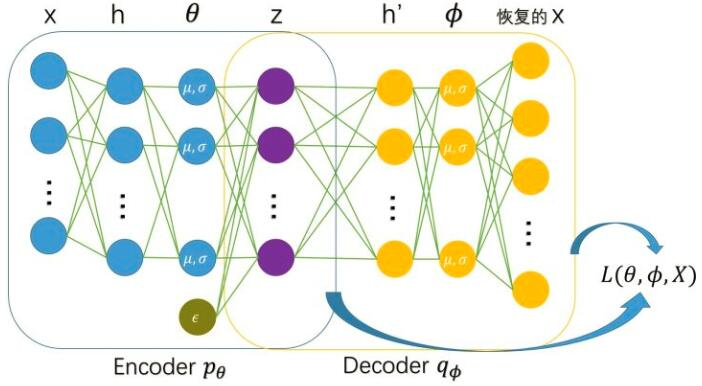# 极简笔记 VAE（变分自编码器）

VAE是一个生成模型，对于生成模型，我们希望求得的都是原始数据分布$p\left(x\right)$$p(x)$。但是我们有的只是离散的对真实分布的采样$\left\{{x}_{1},{x}_{2},{x}_{3},...,{x}_{n}\right\}$$\{x_1,x_2,x_3, ... ,x_n\}$，这就是我们的数据集。这时候我们常常会先假设分布的类型（e.g. 高斯分布，均匀分布），然后用最大似然（ML）来做，计算参数$\theta =\mathrm{arg}\underset{\theta }{max}\sum _{i}\mathrm{log}p\left({x}_{i}\right)$$\theta=\arg\max_{\theta}\sum_i \log p(x_i)$，从而求得${p}_{\theta }\left(x\right)$$p_\theta (x)$。但是$p\left(x\right)$$p(x)$可能是一个很复杂的难以表达的分布，难以选择合适的假设分布来计算最大似然。

$\mathrm{log}{p}_{\theta }\left({x}_{i}\right)$$\log p_\theta(x_i)$有下界$L\left(\theta ,\varphi ;{x}_{i}\right)$$L(\theta,\phi;x_i)$， 这个下界可以进一步拆分：

$L\left(\theta ,\varphi ;{x}_{i}\right)=-{D}_{KL}\left({q}_{\varphi }\left(z|{x}_{i}\right)||{p}_{\theta }\left(z\right)\right)+{\mathbb{E}}_{{q}_{\varphi }\left(z|{x}_{i}\right)}\left[\mathrm{log}{p}_{\theta }\left({x}_{i}|z\right)\right]$

$KL\left(\mathcal{N}\left(\mu ,{\sigma }^{2}\right)||\mathcal{N}\left(0,1\right)\right)$

$=\int \frac{1}{\sqrt{2\pi {\sigma }^{2}}}{e}^{-\left(x-\mu {\right)}^{2}/2{\sigma }^{2}}\left(\mathrm{log}\frac{{e}^{-\left(x-\mu {\right)}^{2}/2{\sigma }^{2}}/\sqrt{2\pi {\sigma }^{2}}}{{e}^{-{x}^{2}/2{\sigma }^{2}}/\sqrt{2\pi }}\right)dx$

$=\int \frac{1}{\sqrt{2\pi {\sigma }^{2}}}{e}^{-\left(x-\mu {\right)}^{2}/2{\sigma }^{2}}\mathrm{log}\left(\frac{1}{\sqrt{{\sigma }^{2}}}exp\left(\frac{1}{2}\left[{x}^{2}-\left(x-\mu {\right)}^{2}/{\sigma }^{2}\right]\right)\right)dx\phantom{\rule{0ex}{0ex}}$

$=\frac{1}{2}\int \frac{1}{\sqrt{2\pi {\sigma }^{2}}}{e}^{-\left(x-\mu {\right)}^{2}/2{\sigma }^{2}}\left[-\mathrm{log}{\sigma }^{2}+{x}^{2}-\left(x-\mu {\right)}^{2}/{\sigma }^{2}\right]dx$

$=\frac{1}{2}\left(-\mathrm{log}{\sigma }^{2}+{\mu }^{2}+{\sigma }^{2}-1\right)$${\mathbb{E}}_{{q}_{\varphi }\left(z|{x}_{i}\right)}\left[\mathrm{log}{p}_{\theta }\left({x}_{i}|z\right)\right]=\frac{1}{L}\sum _{l=1}^{L}\left(\mathrm{log}{p}_{\theta }\left({x}_{i}|{z}_{i,l}\right)\right)$

$L\left(\theta ,\varphi ;{x}_{i}\right)\simeq \frac{1}{2}\sum _{j=1}^{J}\left(1+\mathrm{log}\left(\left({\sigma }_{i}^{j}{\right)}^{2}\right)-\left({\mu }_{i}^{j}{\right)}^{2}-\left({\sigma }_{i}^{j}{\right)}^{2}\right)+\frac{1}{L}\sum _{l=1}^{L}\mathrm{log}{p}_{\theta }\left({x}_{i}|{z}_{i,l}\right)$